# RS Aggarwal Solutions for Class 8 Maths Chapter 1 - Rational Numbers Exercise 1C

RS Aggarwal Solutions for Class 8 Maths Chapter 1- Exercise 1C, Rational Numbers are prepared by our BYJU’s expert team, where students can download from the links provided below.

In Exercise 1C of RS Aggarwal Class 8 Maths, we shall study the Addition and Subtraction of Rational Numbers and Properties of Addition of Rational Numbers.

In properties, we see Closure property, Commutative Law, Associative Law, Existence of Additive Identity, Existence of Additive Inverse.

## Download PDF of RS Aggarwal Solutions for Class 8 Maths Chapter 1- Rational Numbers Exercise 1C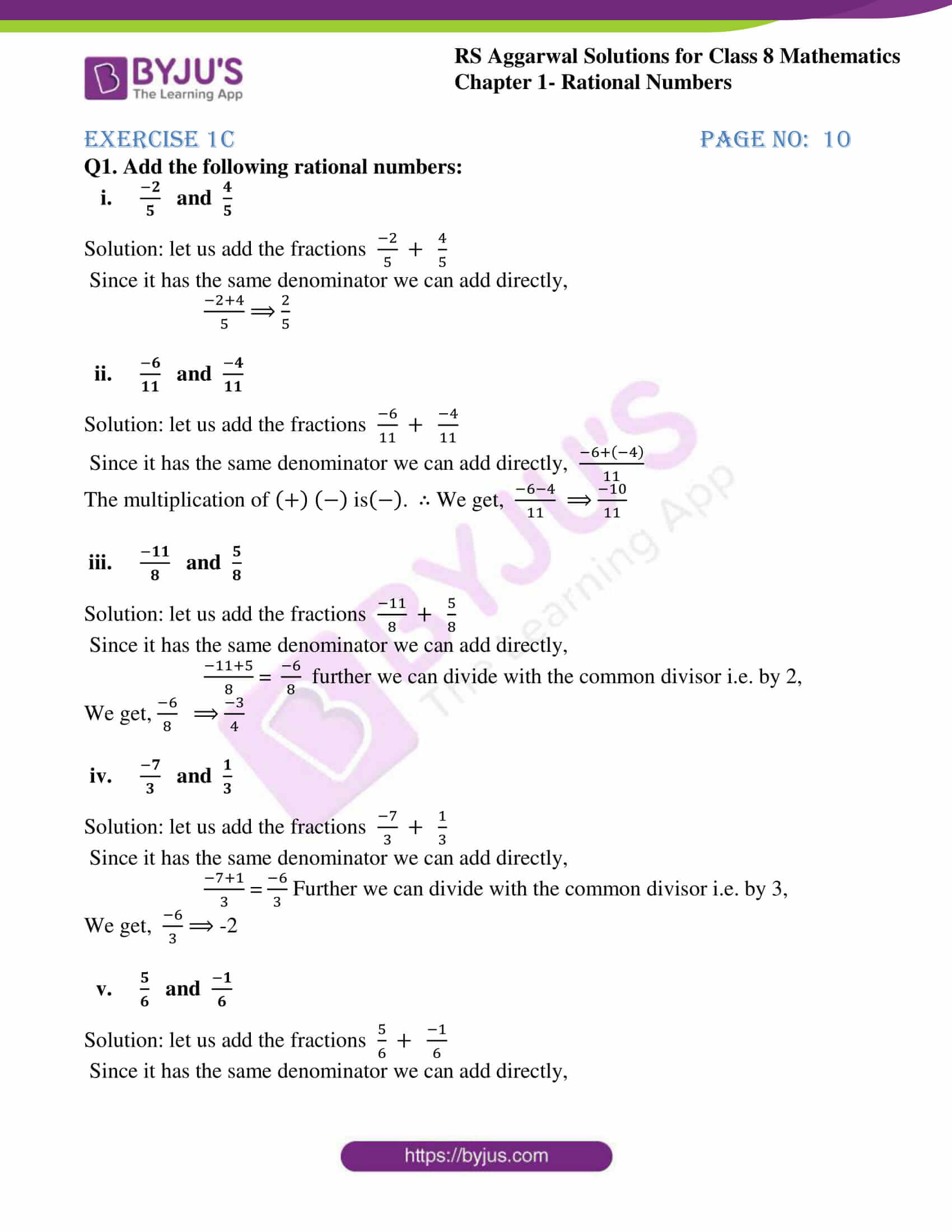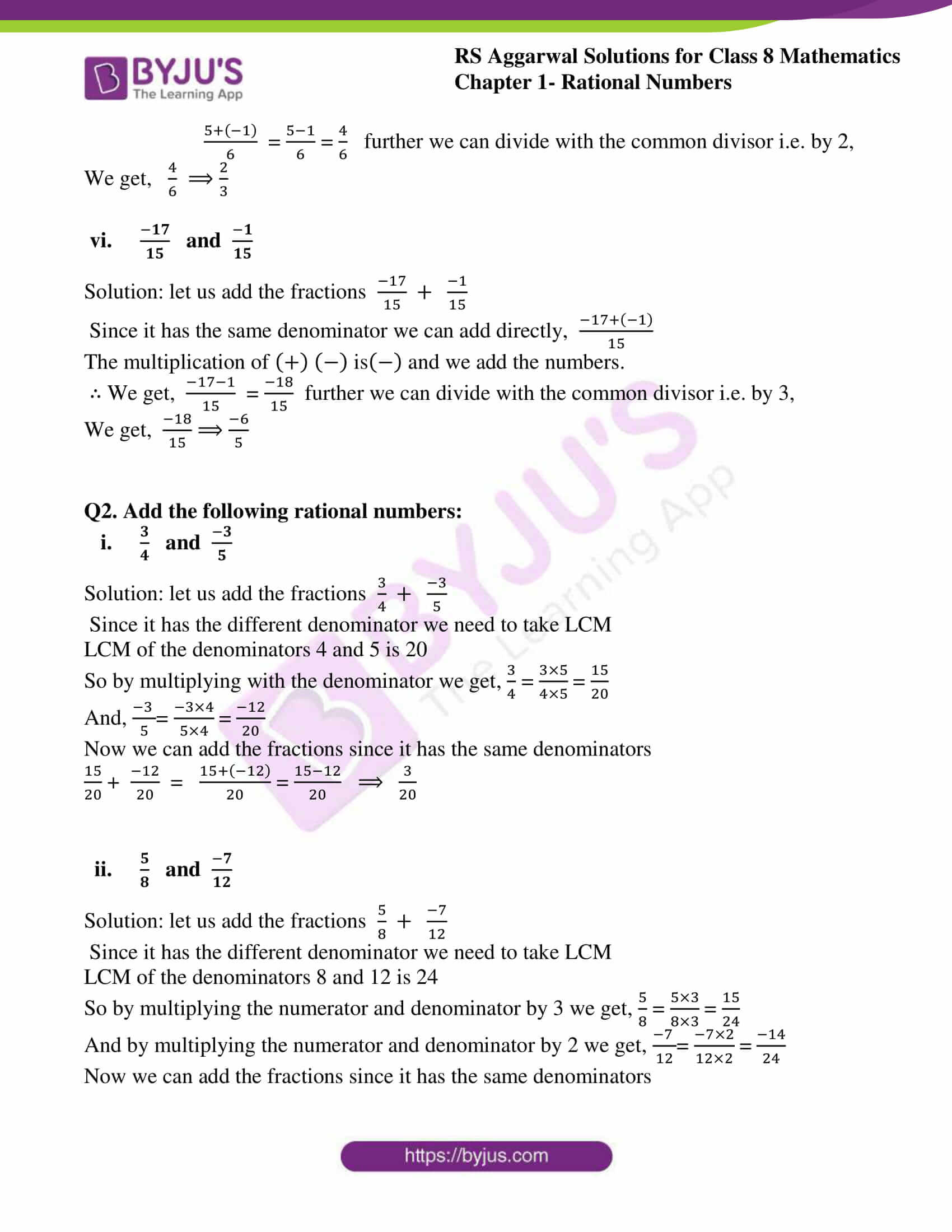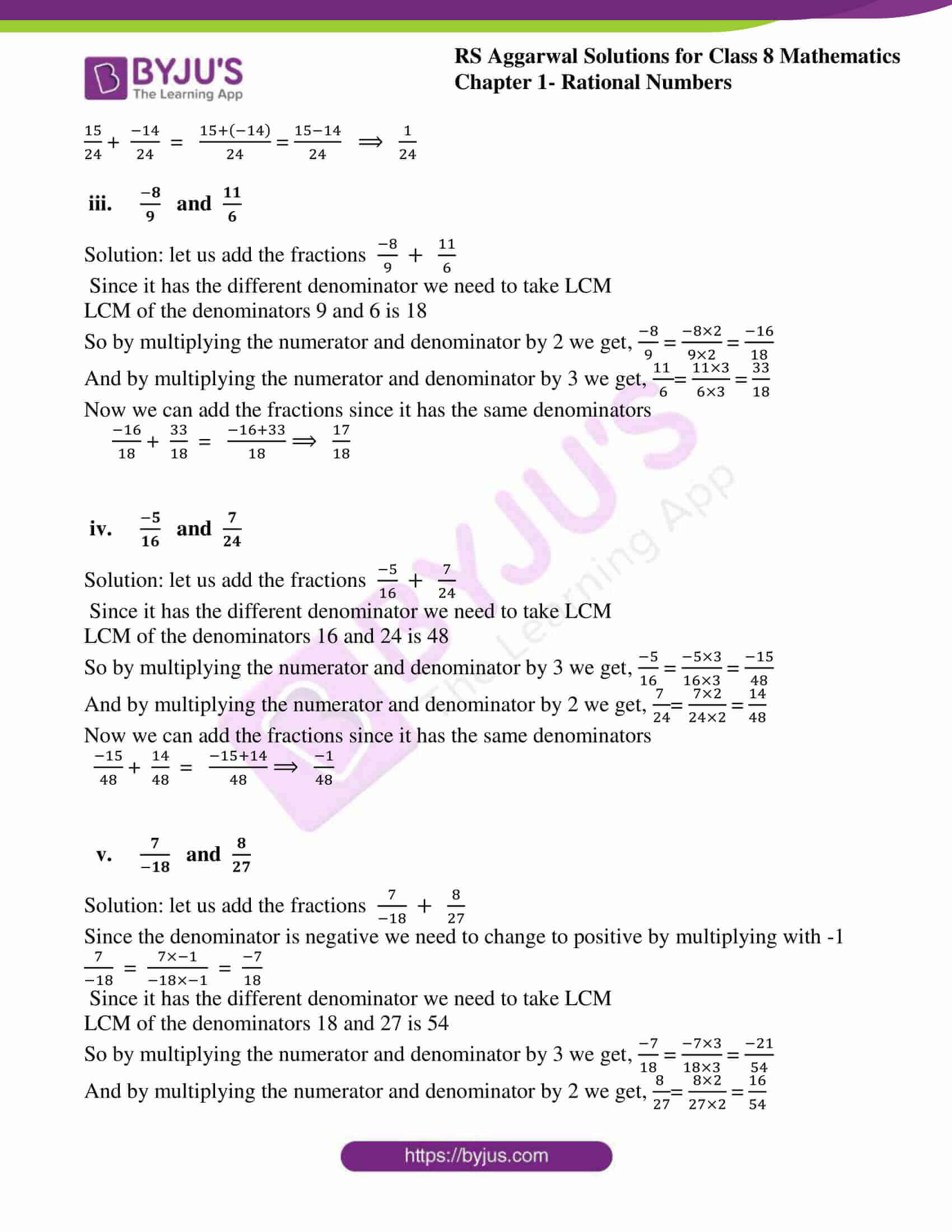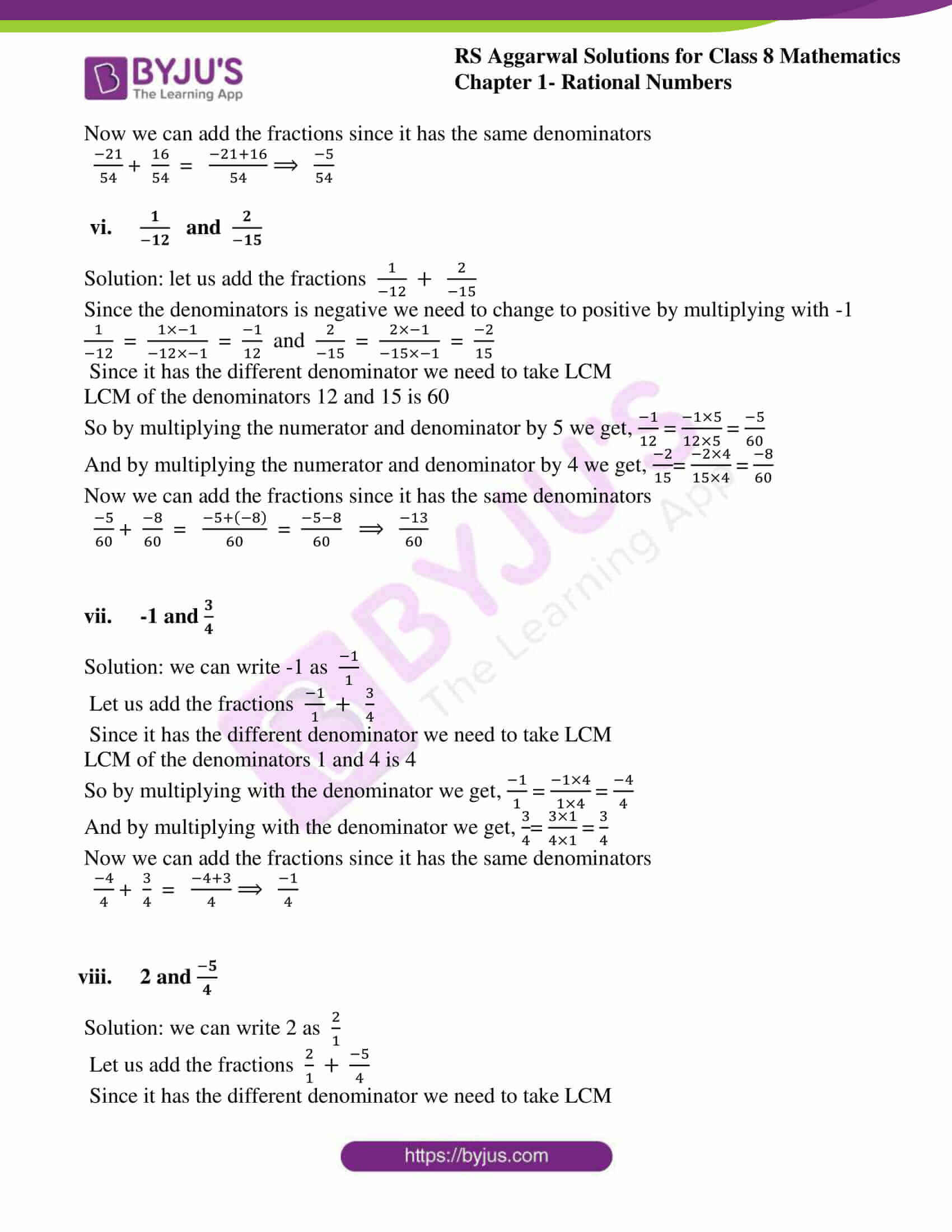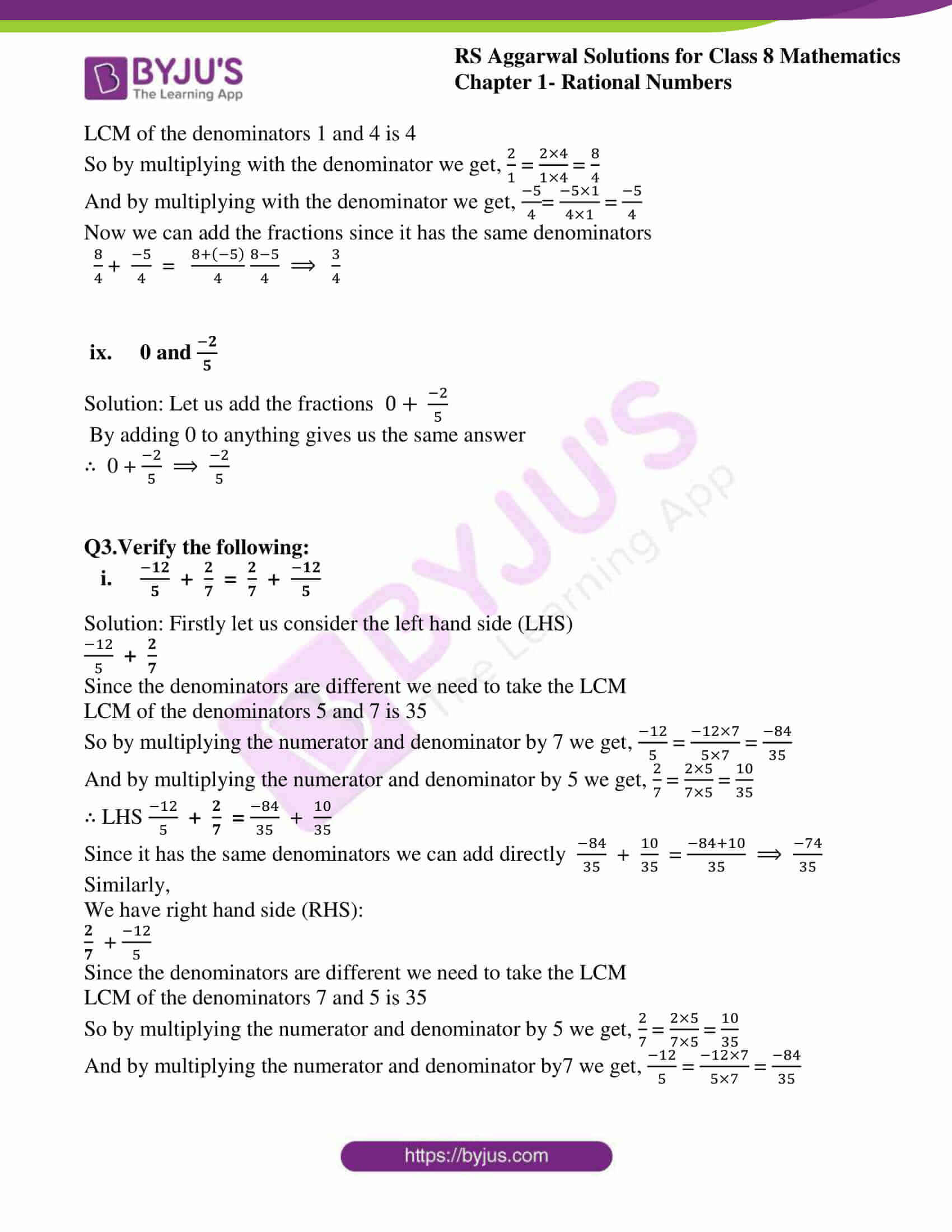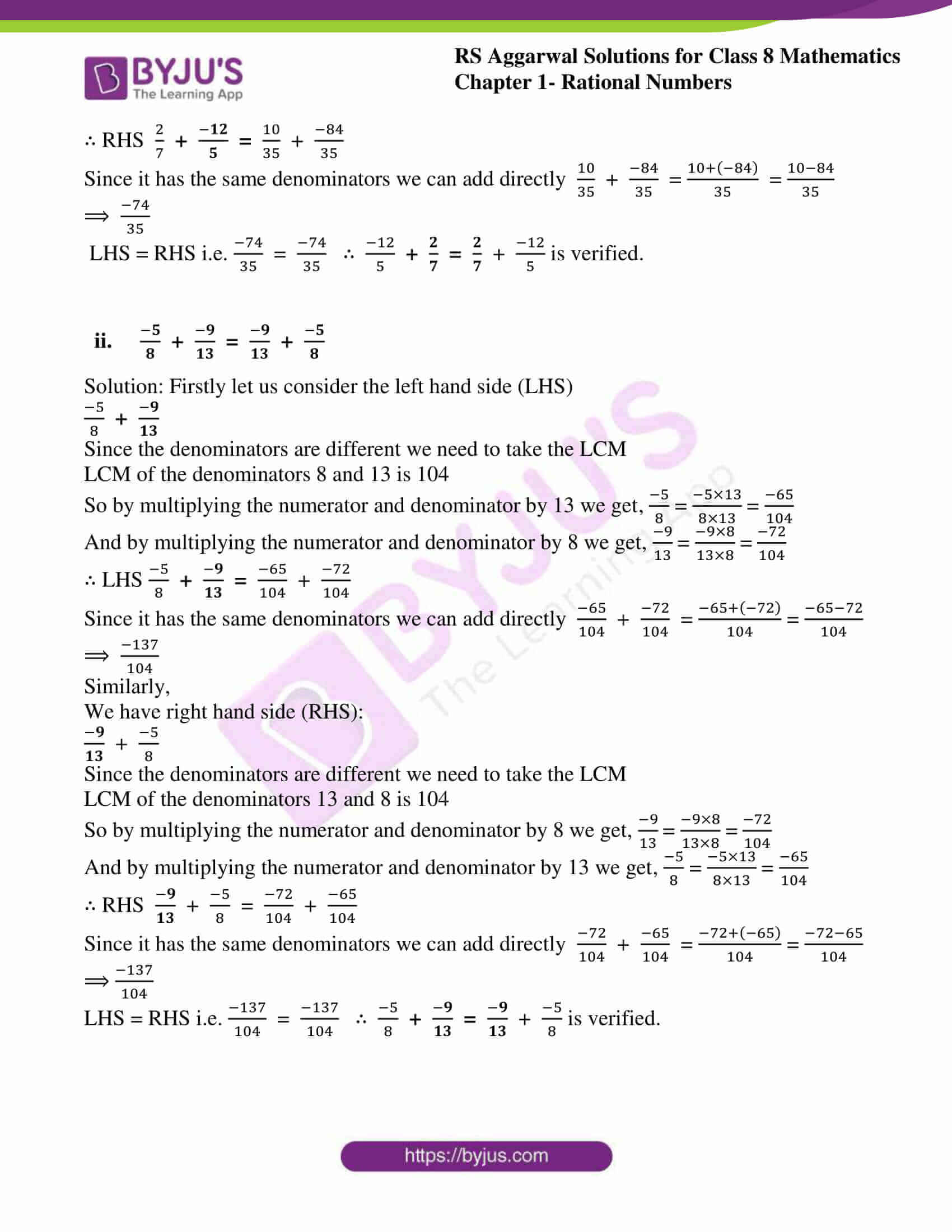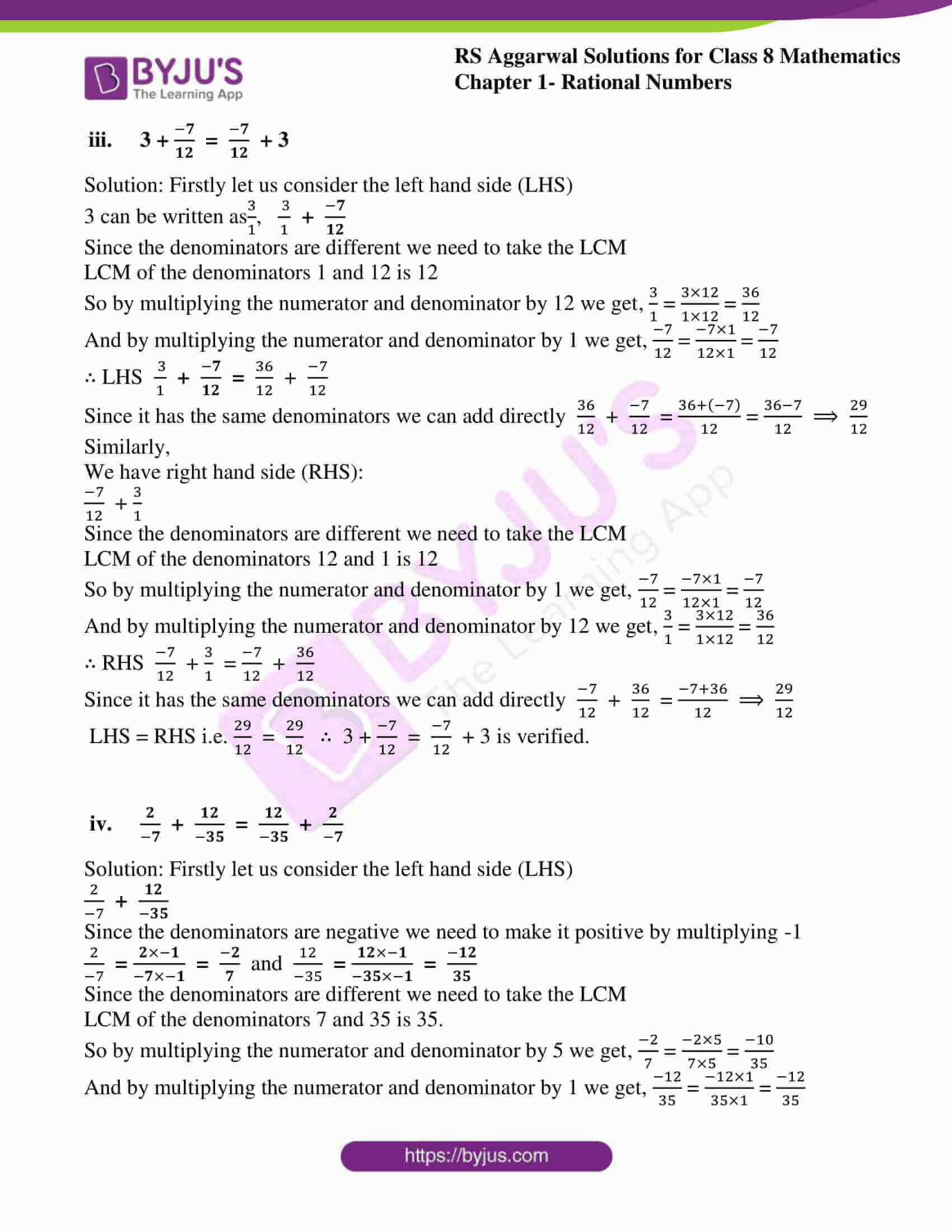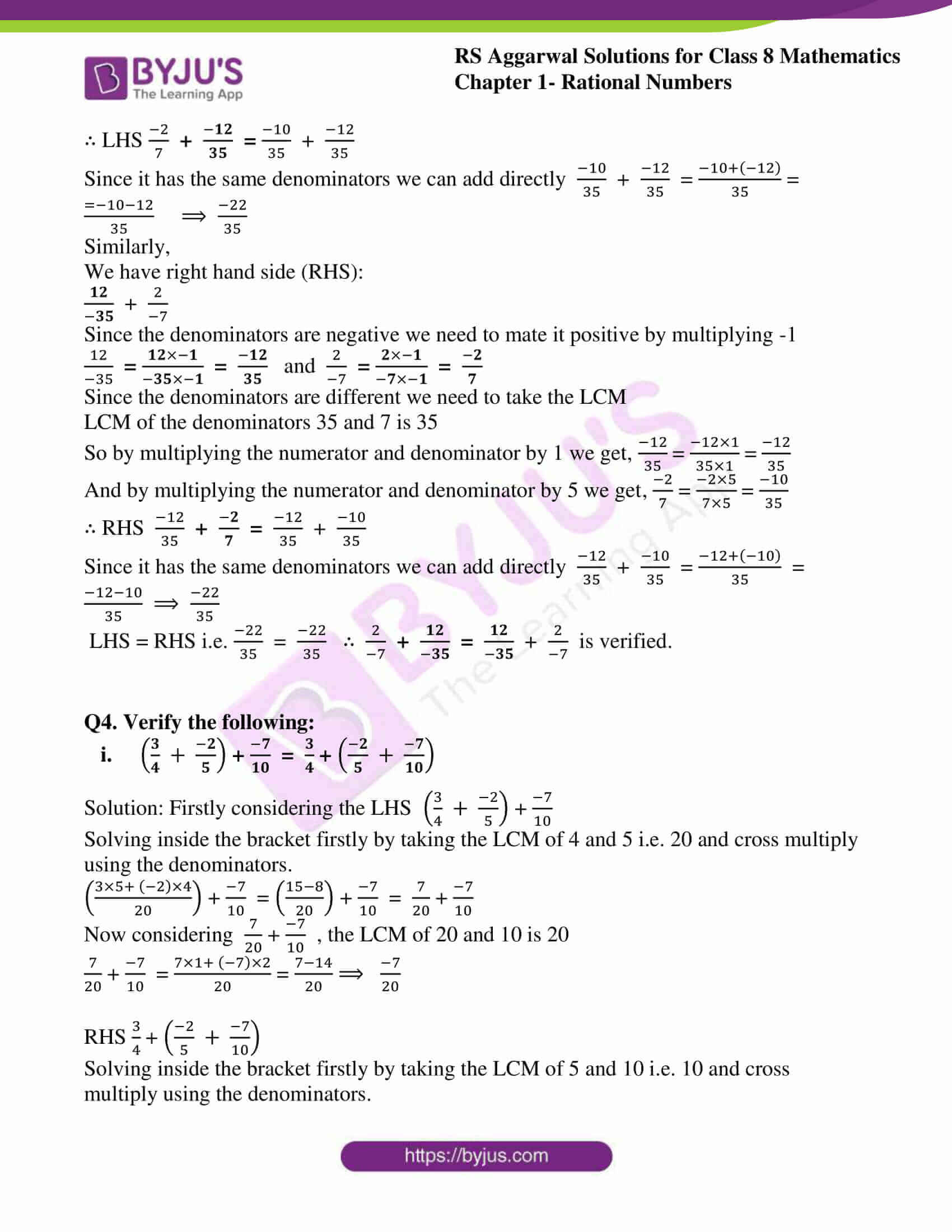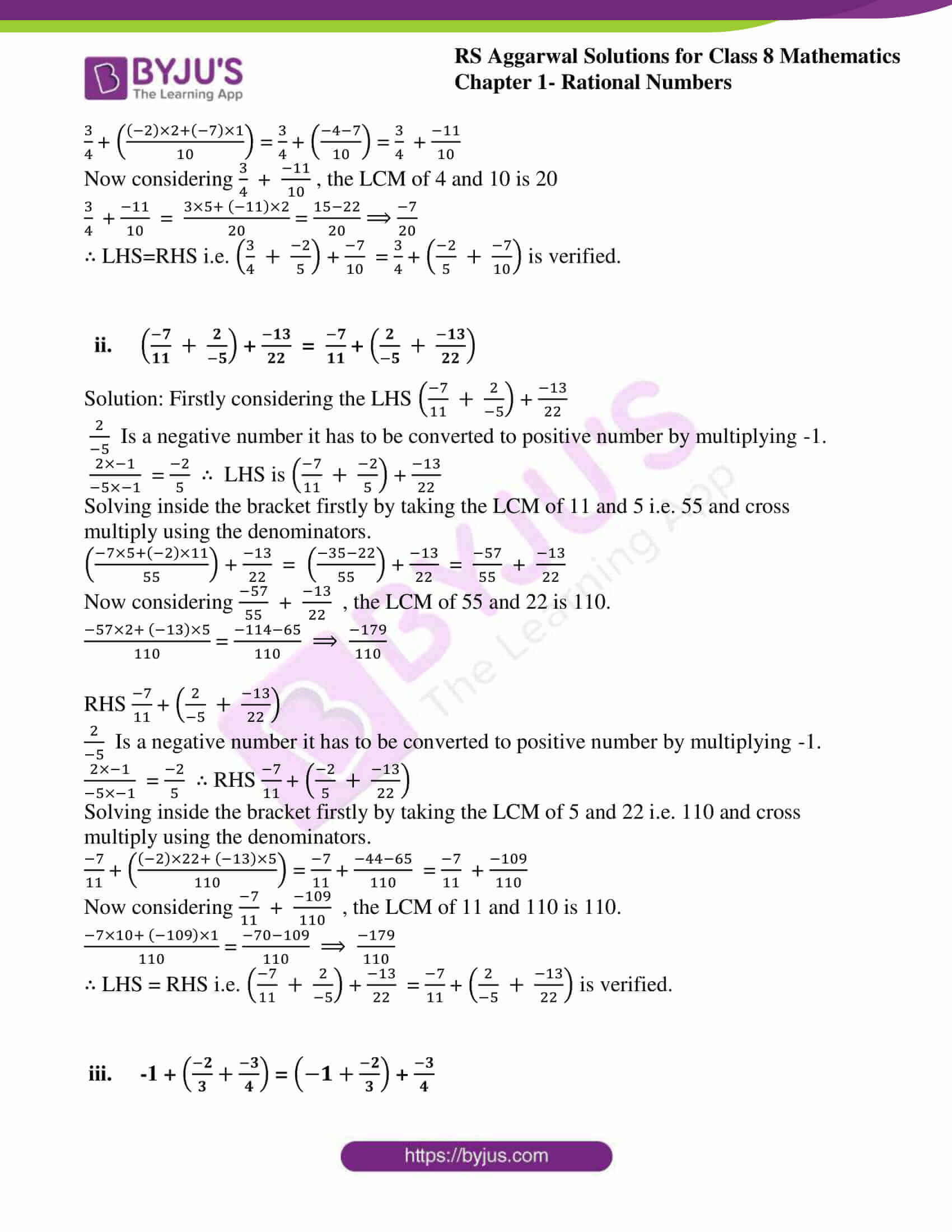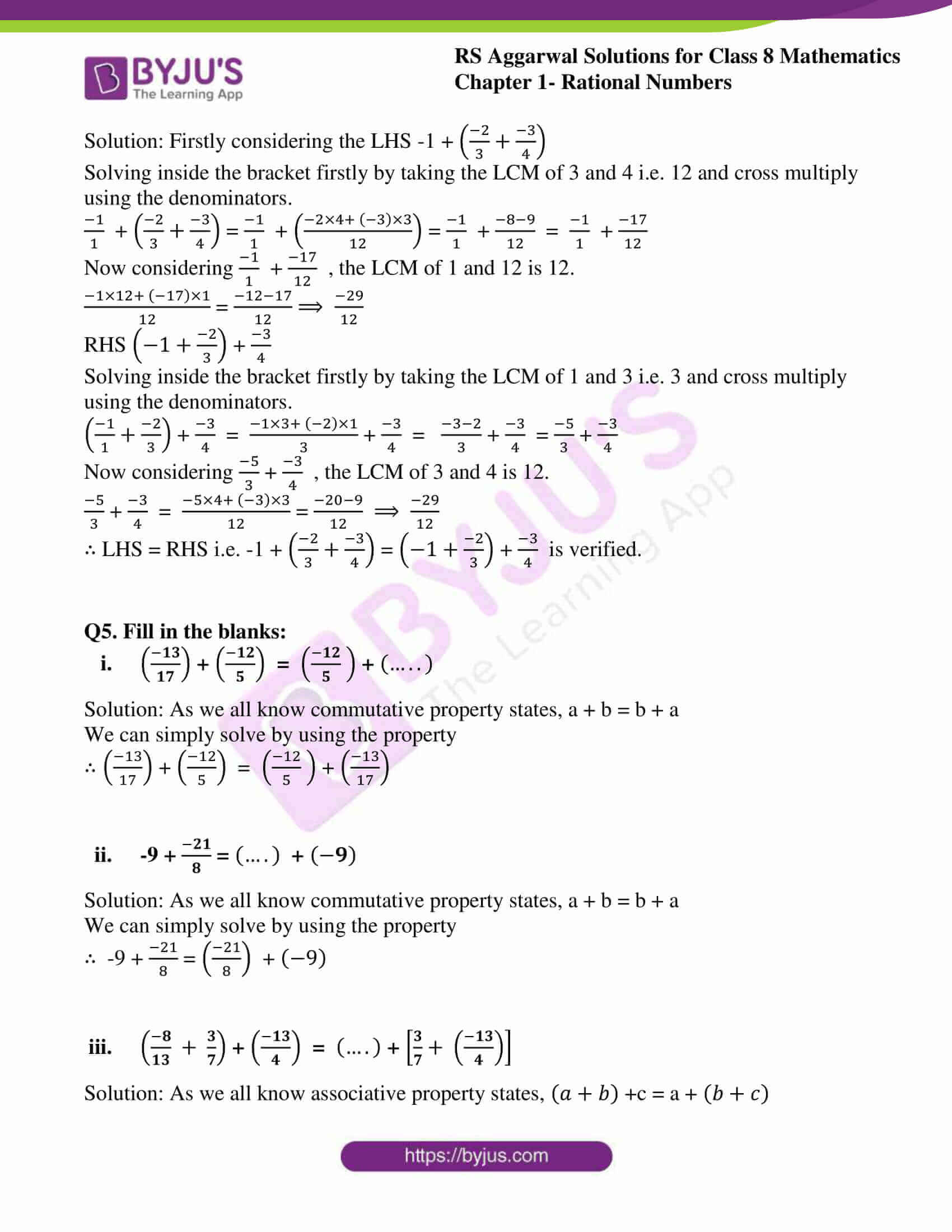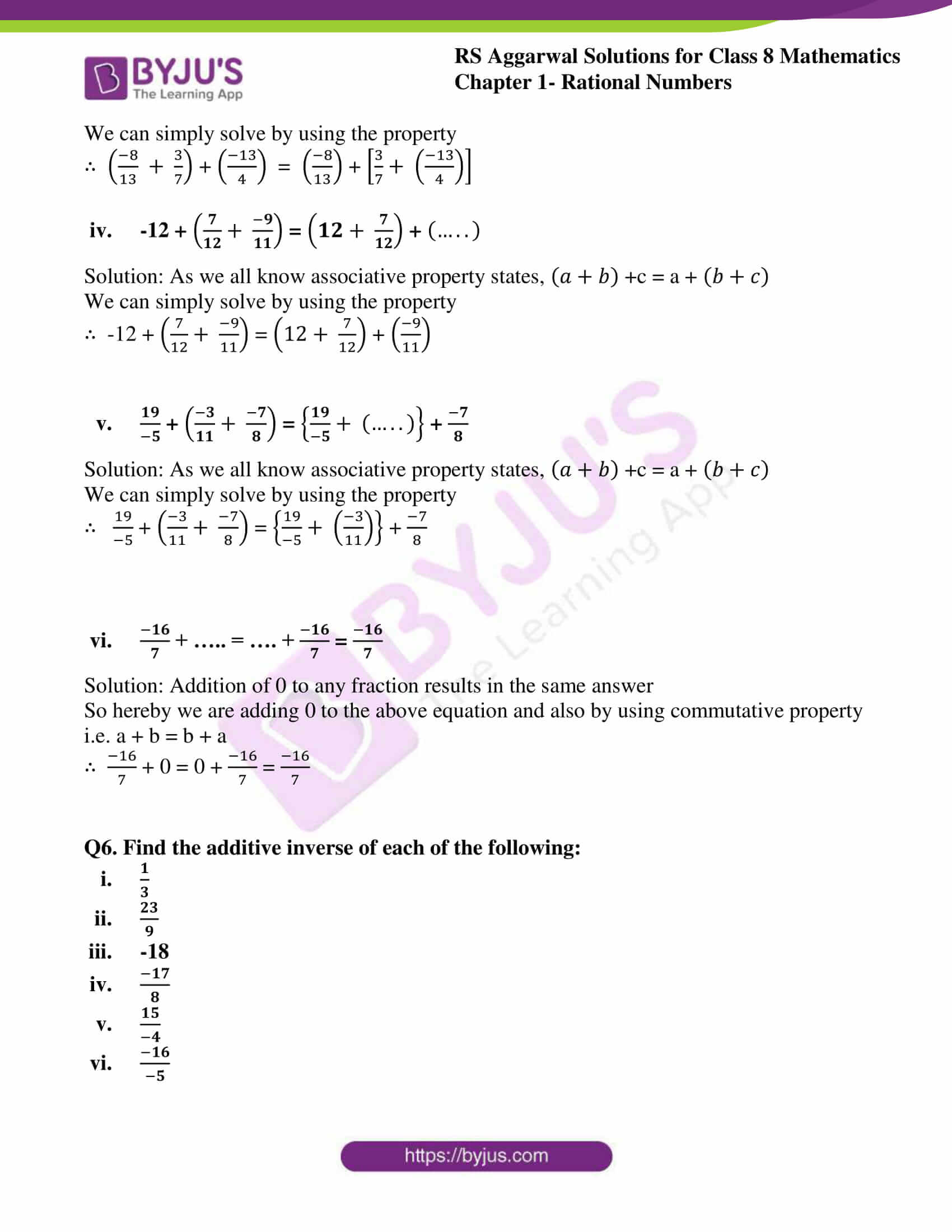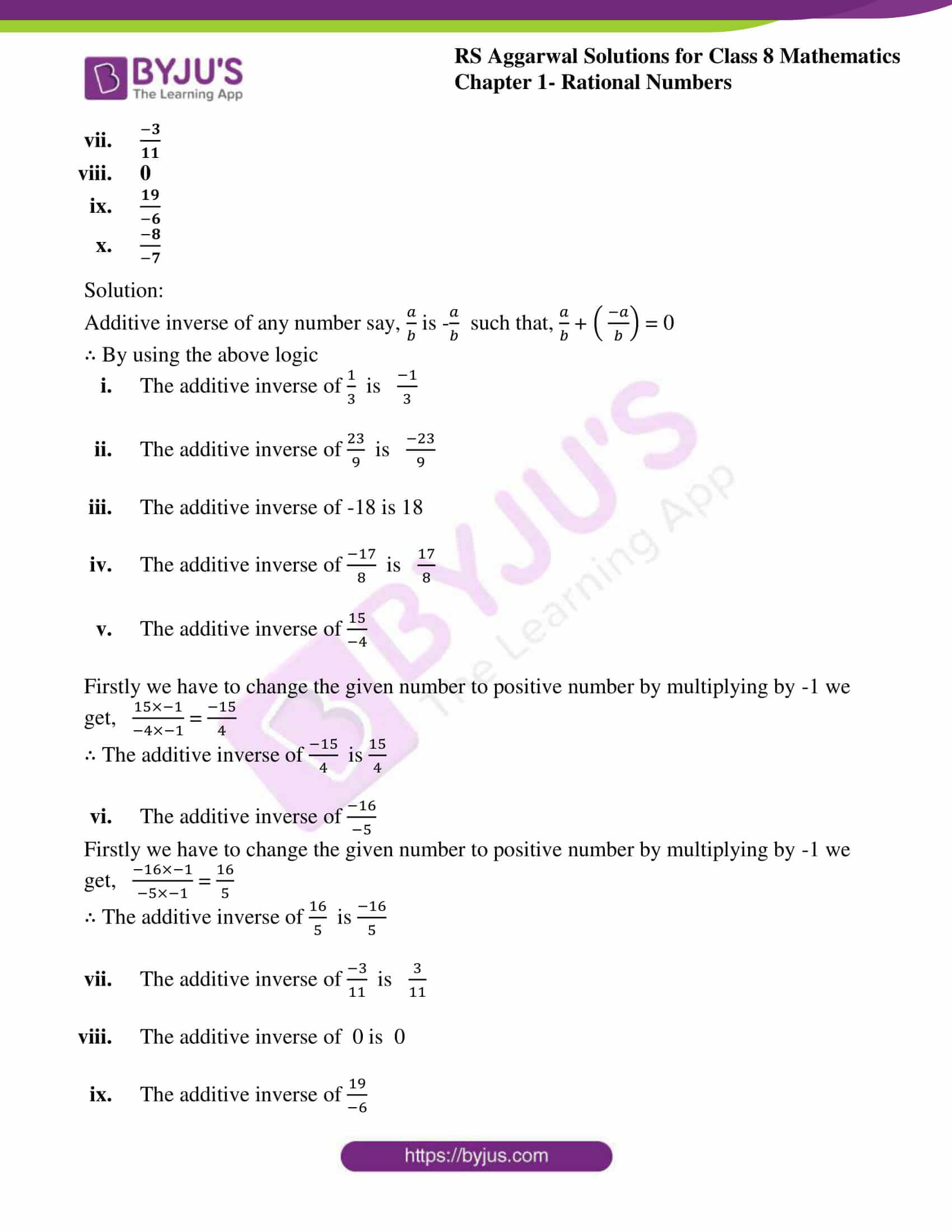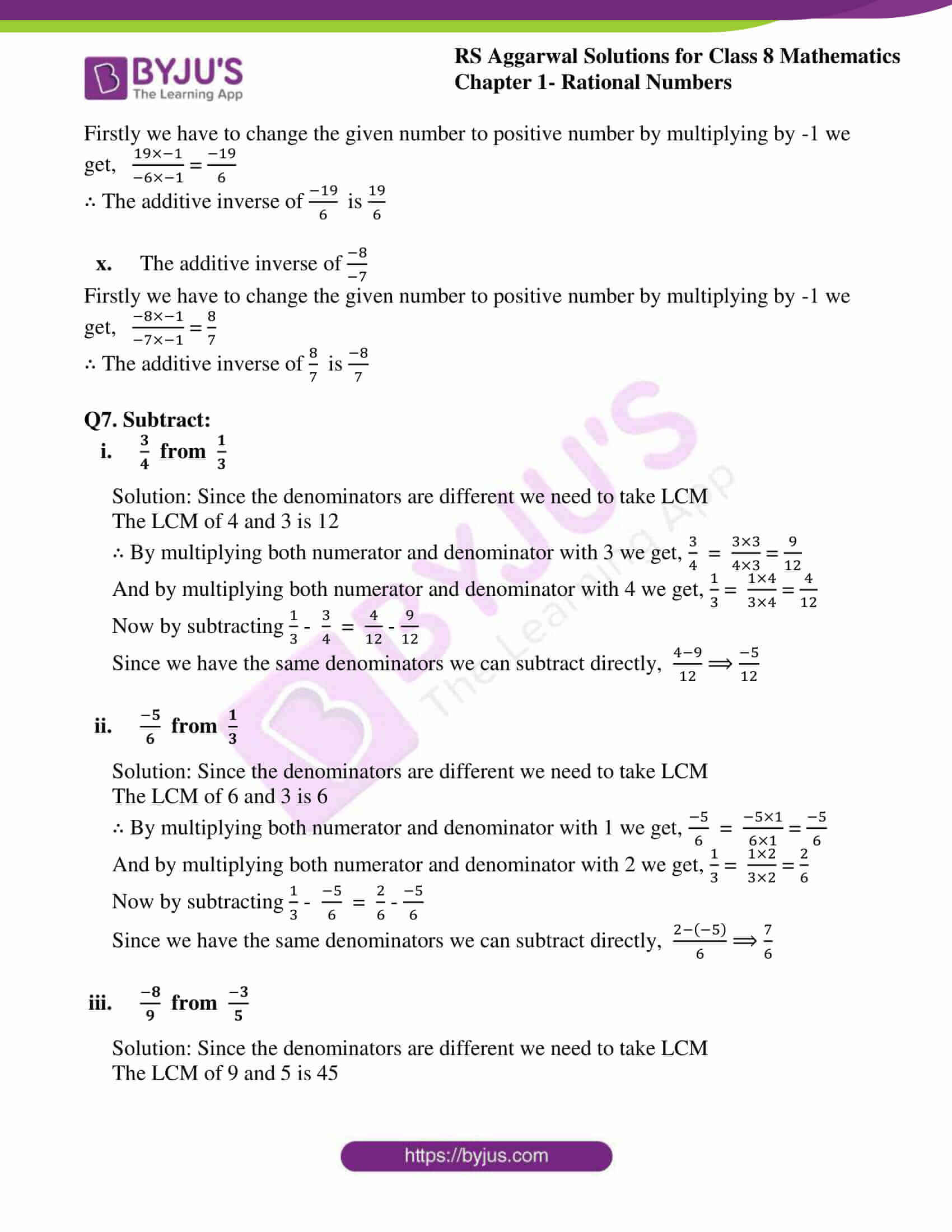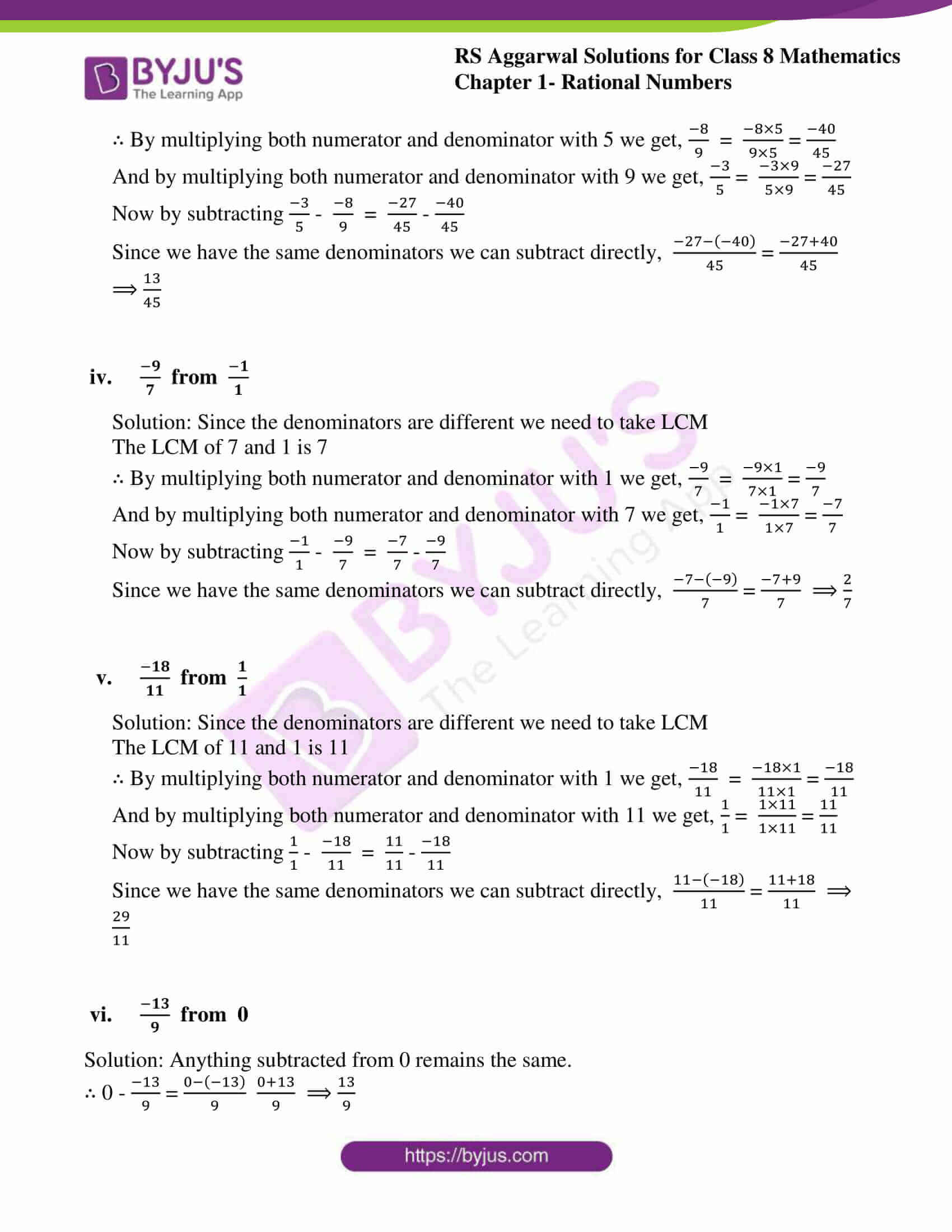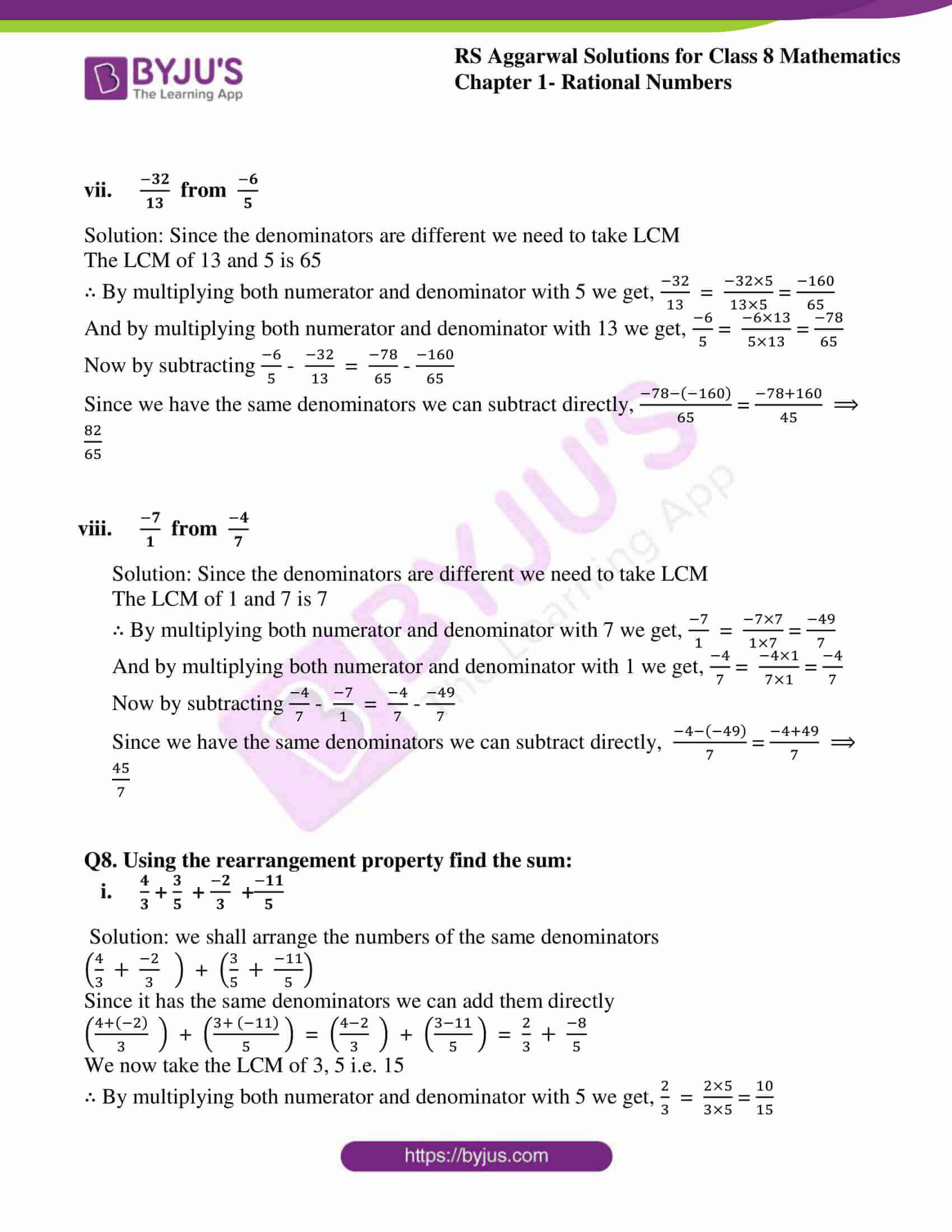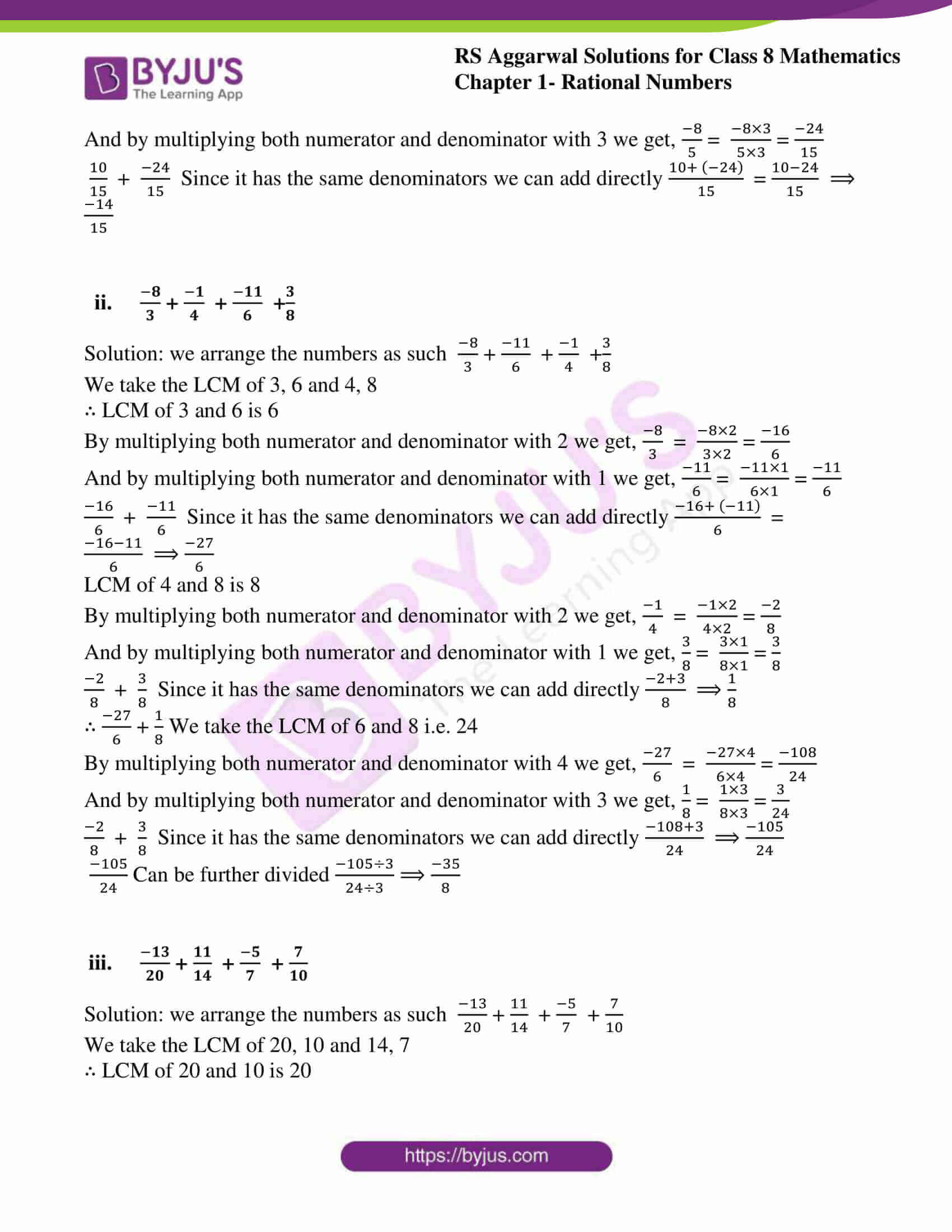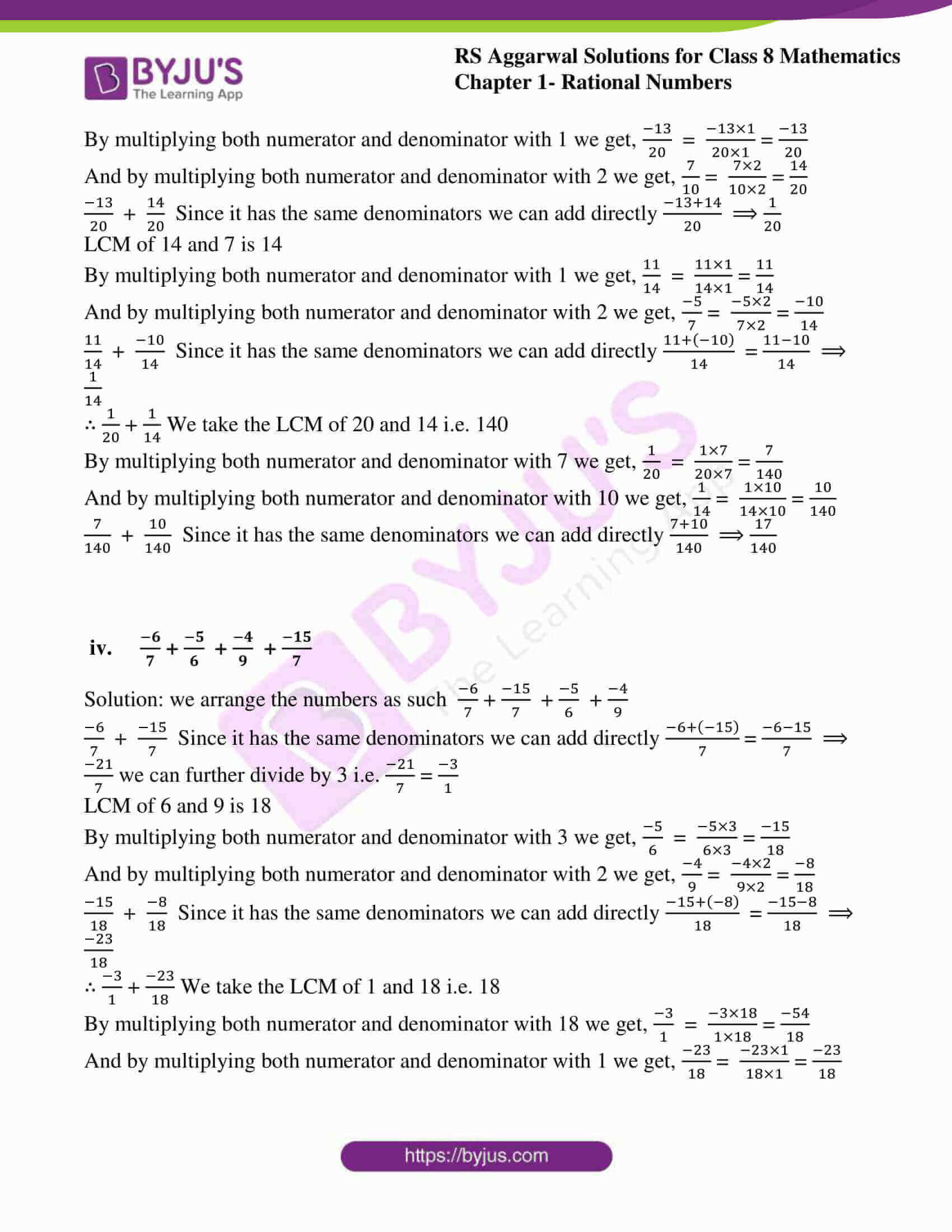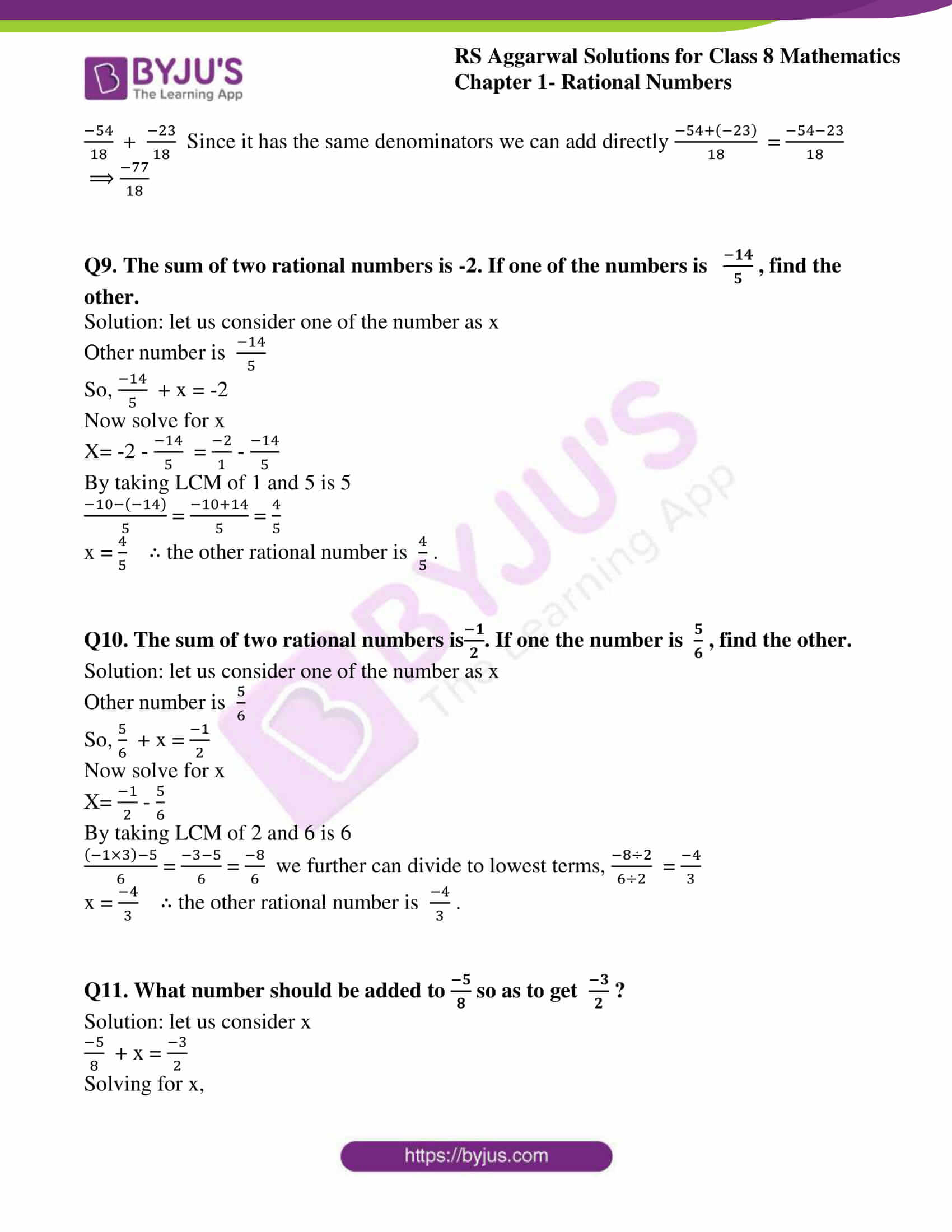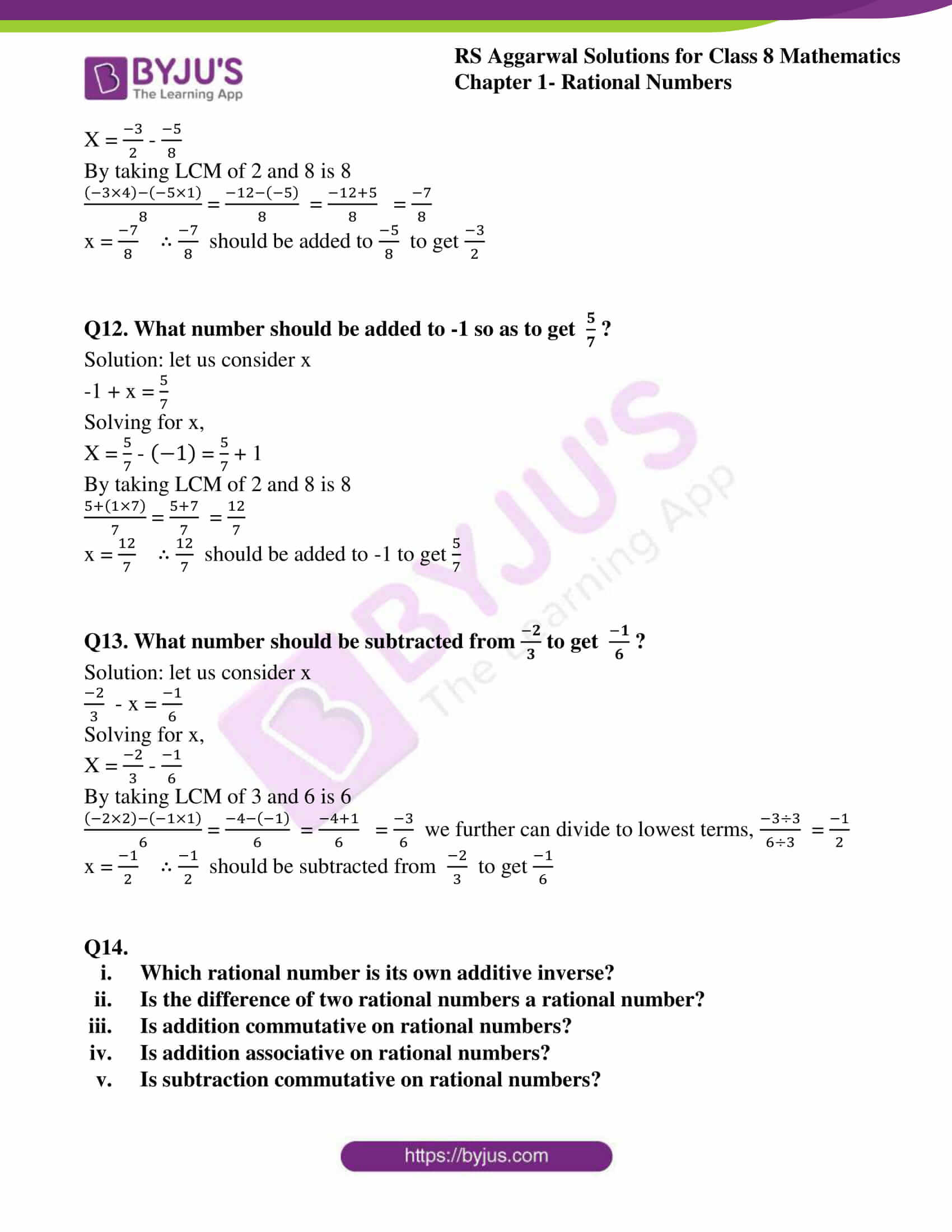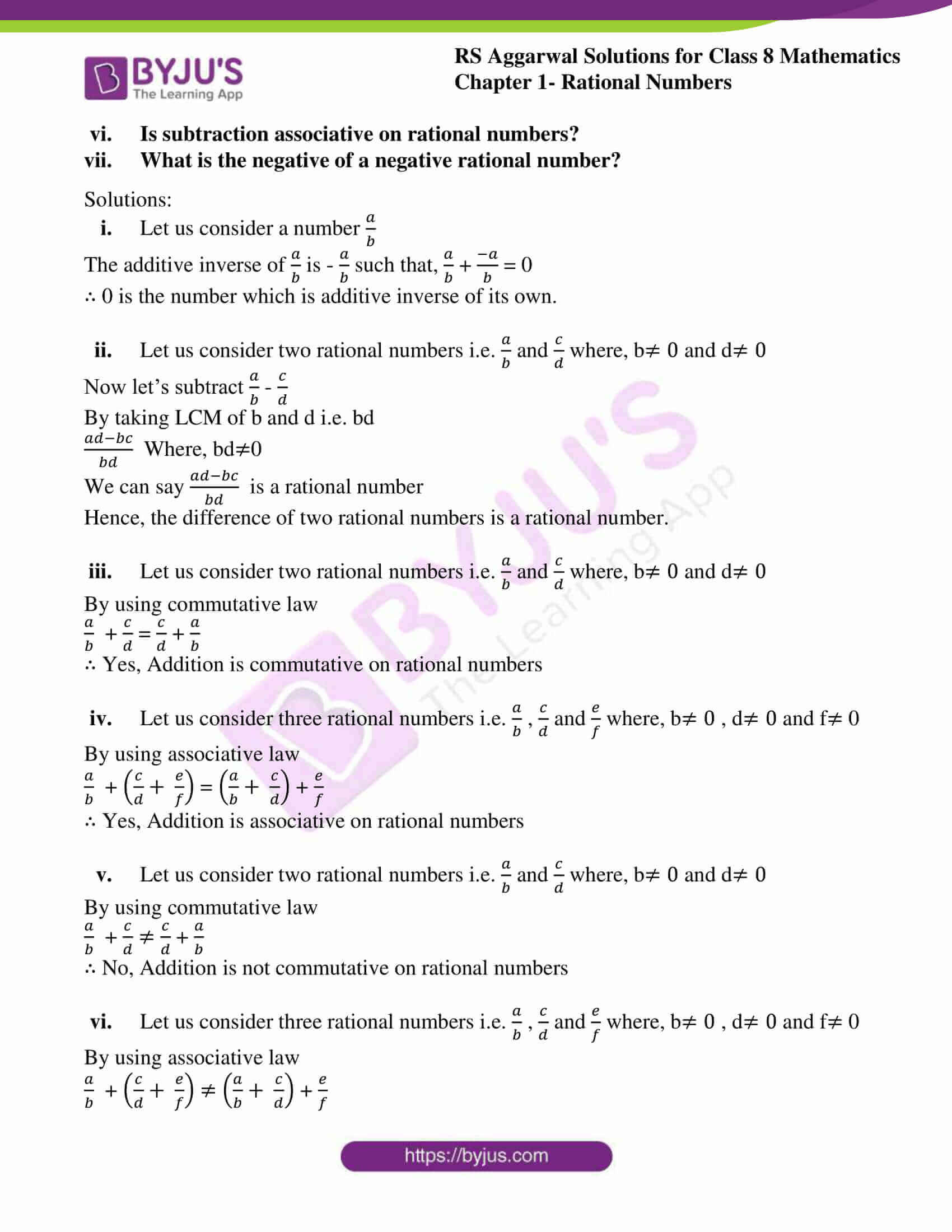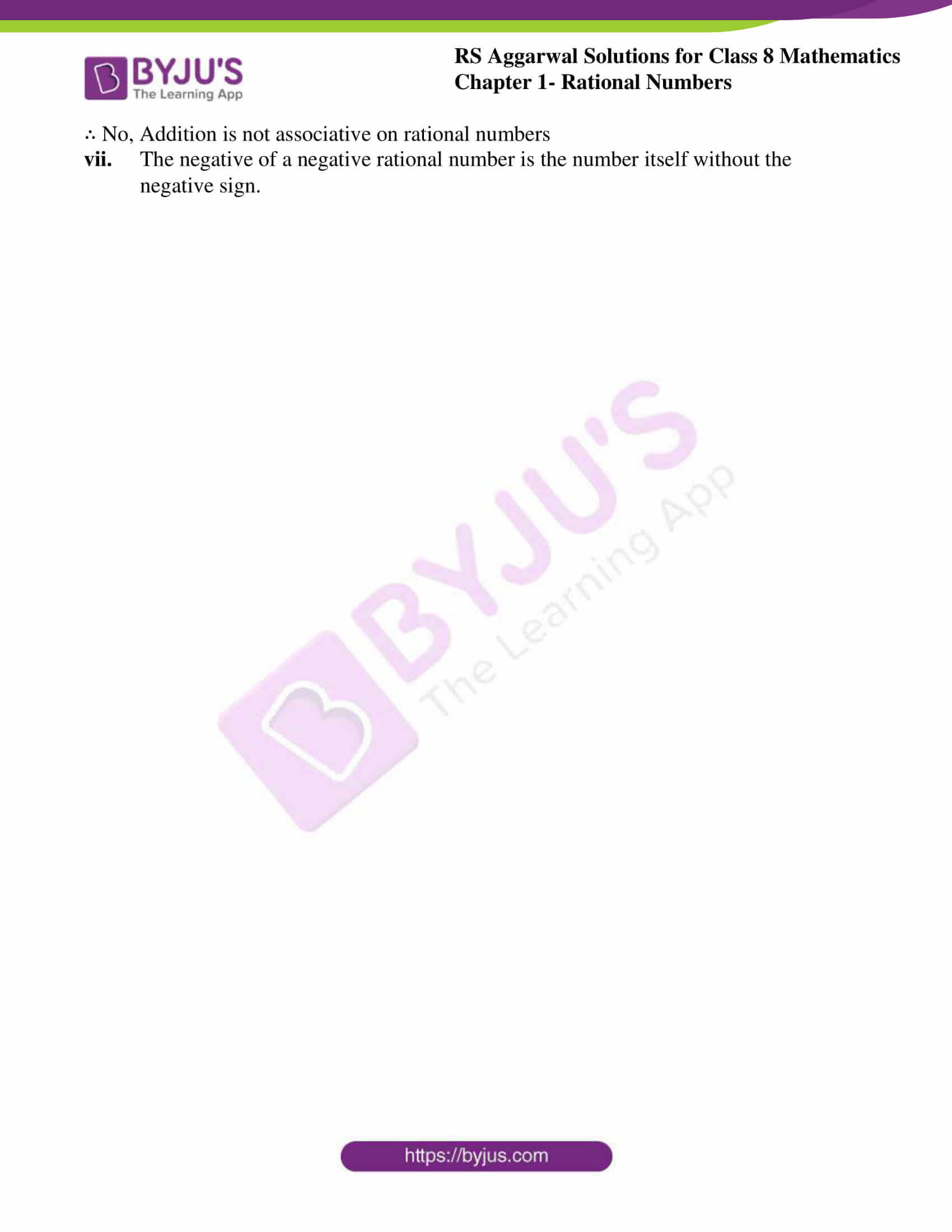### Access Answers to RS Aggarwal Solutions for Class 8 Maths Chapter 1- Rational Numbers Exercise 1C

Q1. Add the following rational numbers: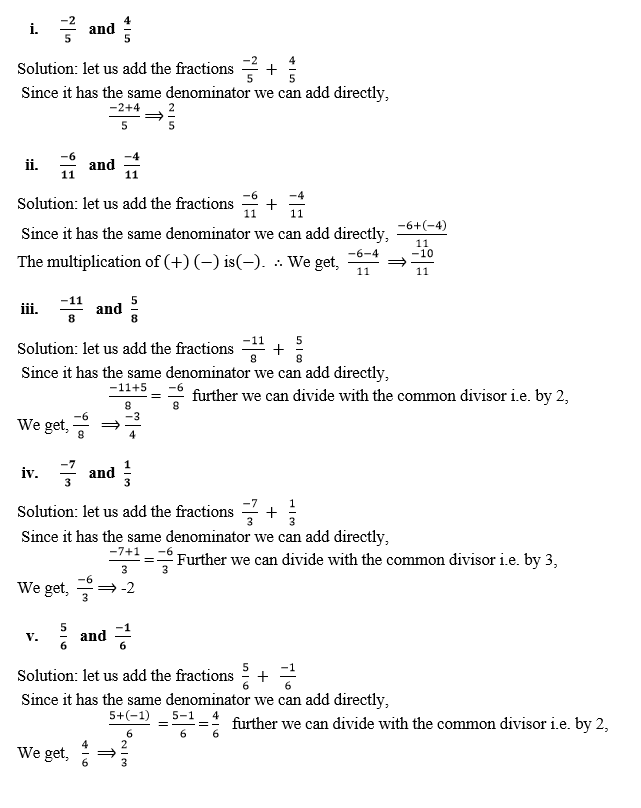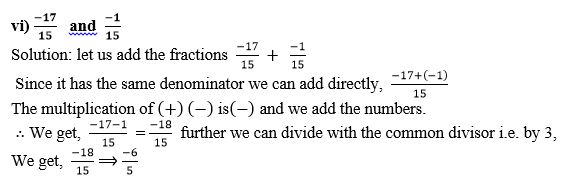Q2. Add the following rational numbers: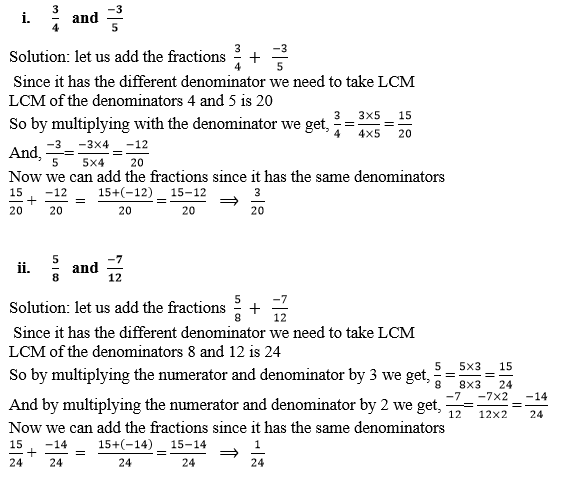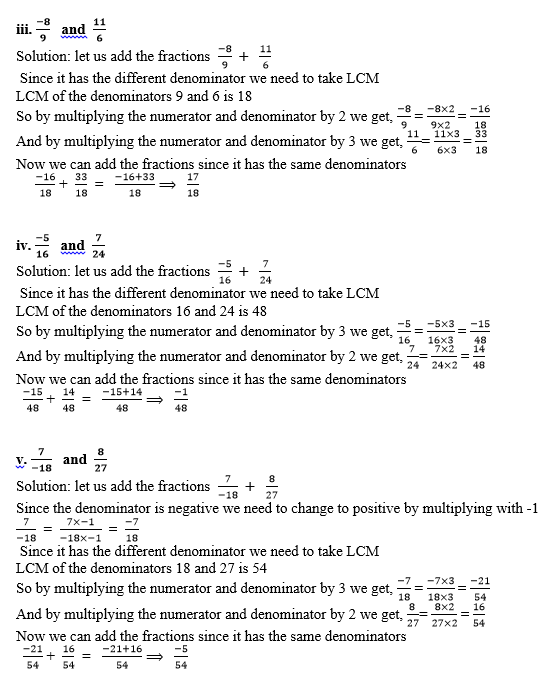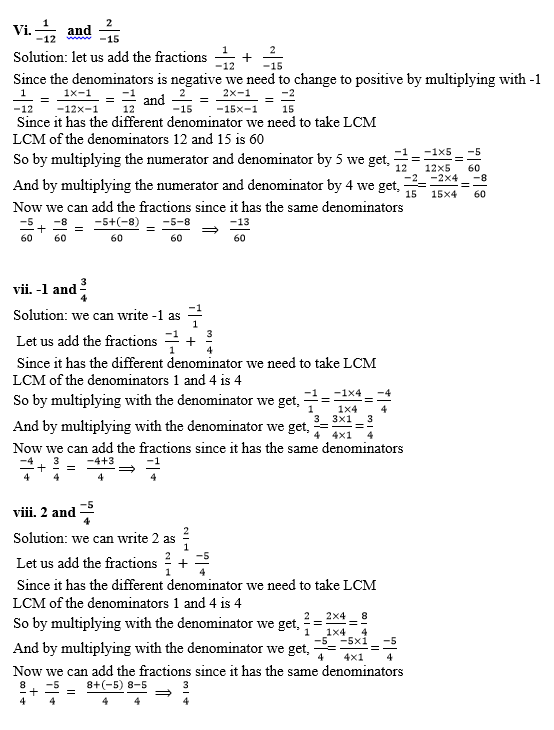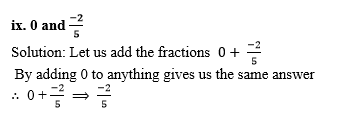Q3.Verify the following: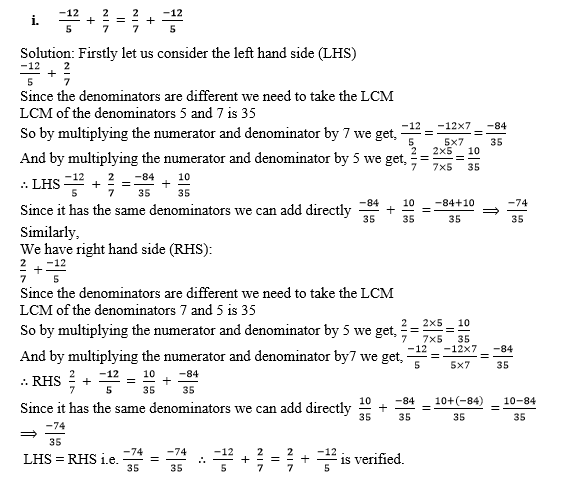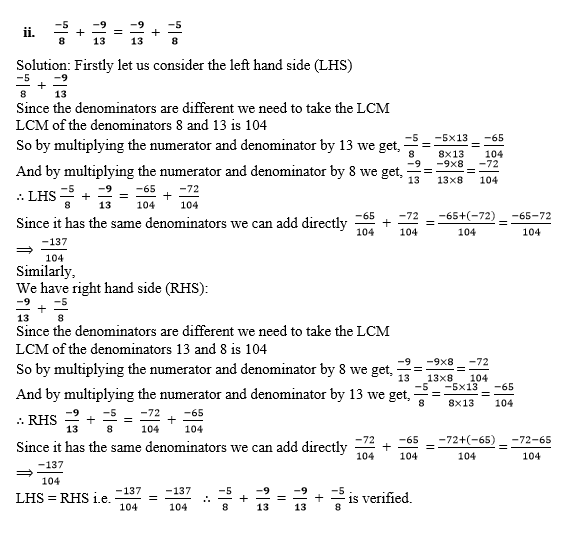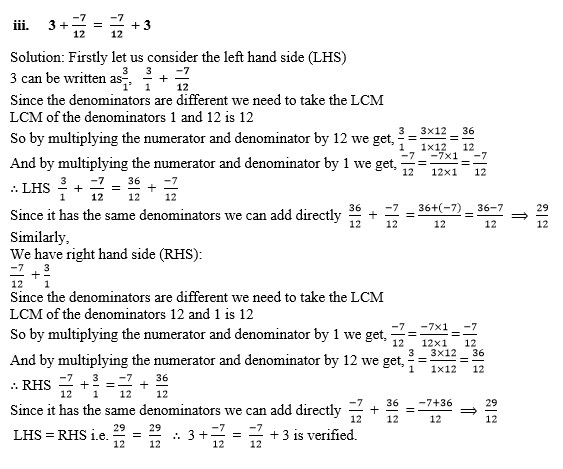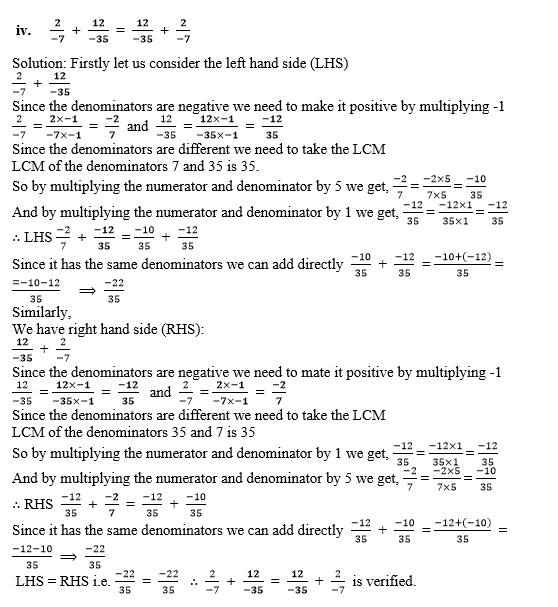Q4. Verify the following: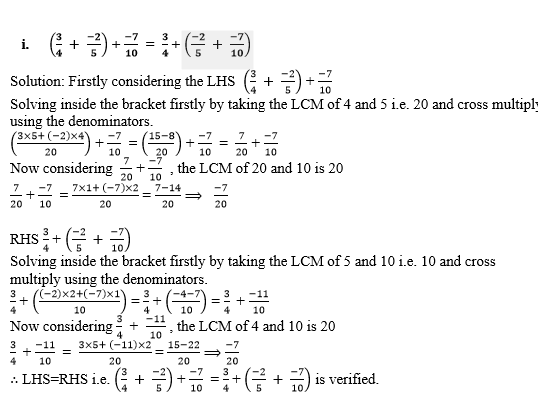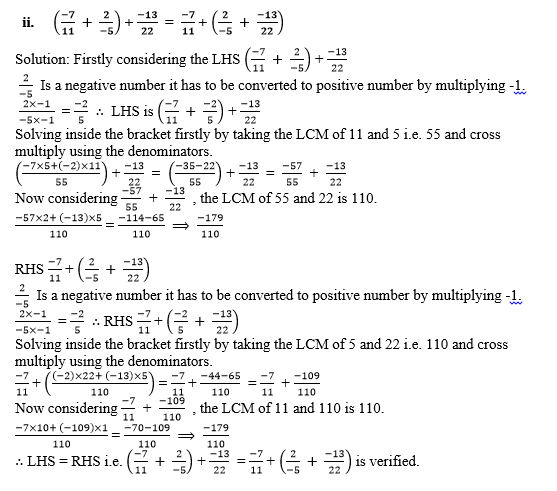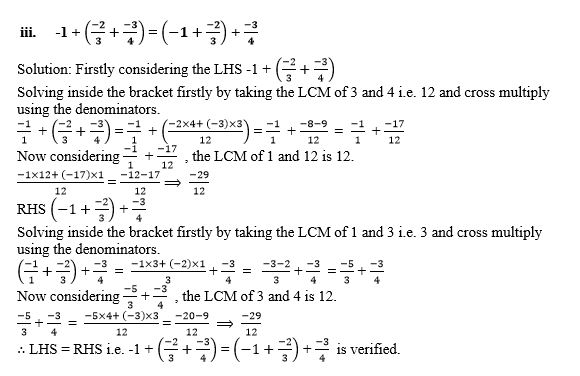Q5. Fill in the blanks: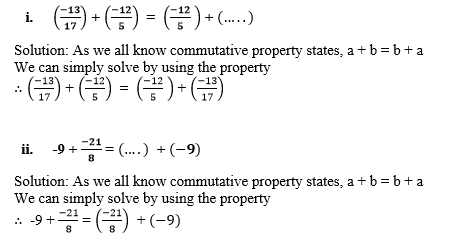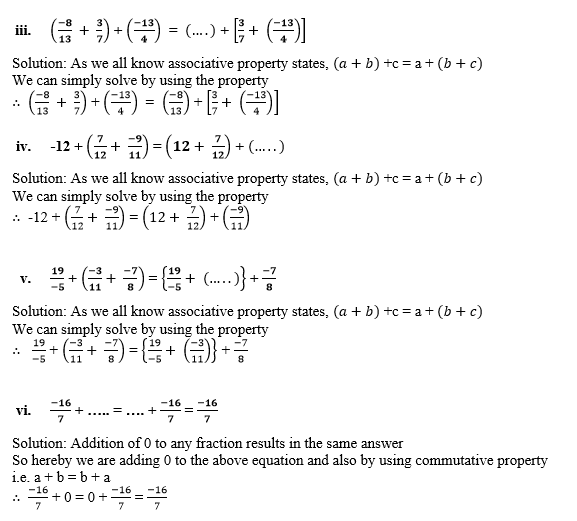Q6. Find the additive inverse of each of the following: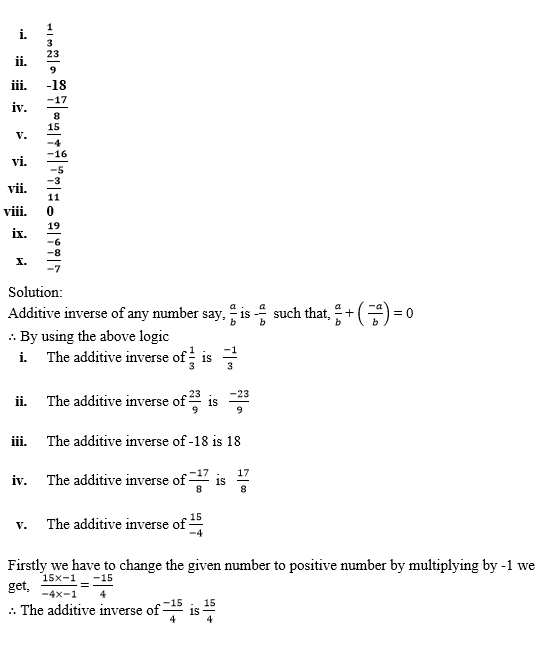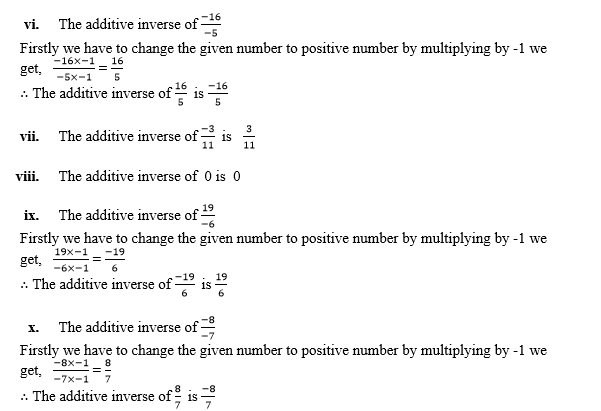Q7. Subtract: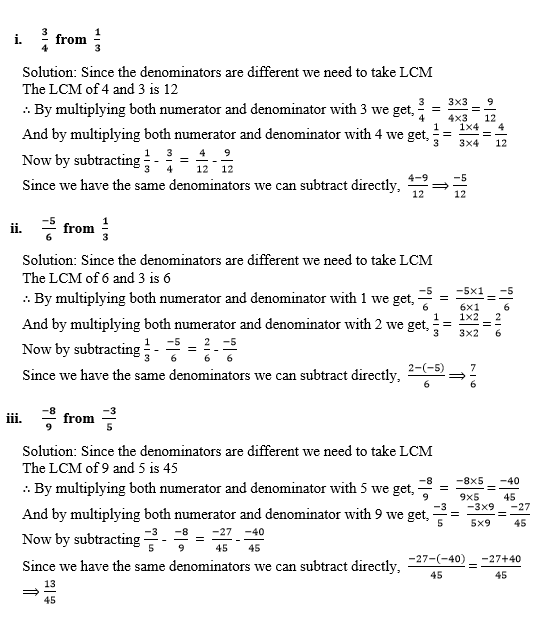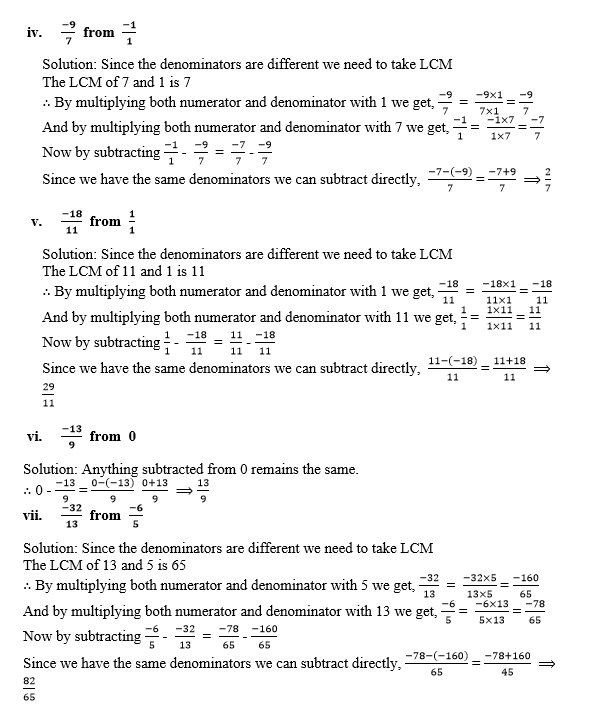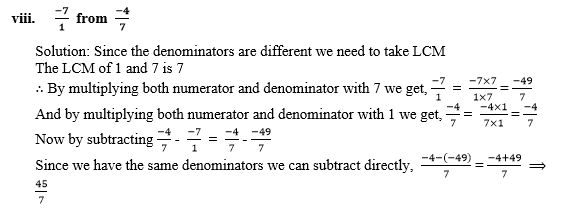Q8. Using the rearrangement property find the sum: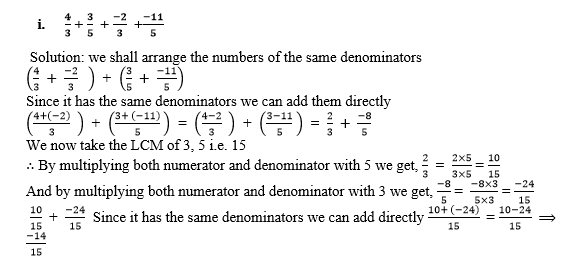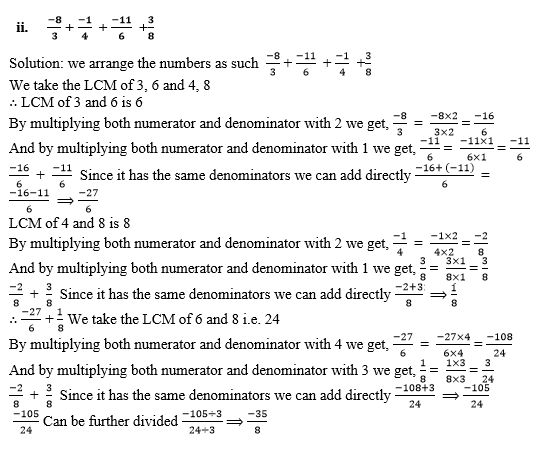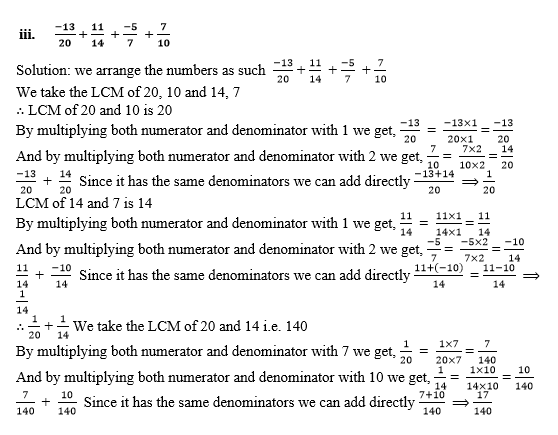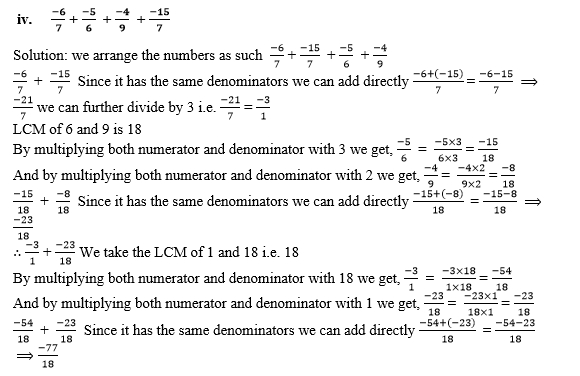Q9. The sum of two rational numbers is -2. If one of the numbers is -14/5 , find the other.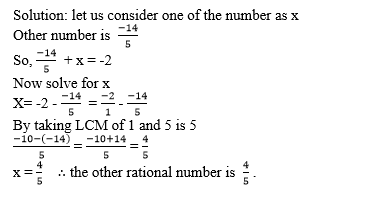Q10. The sum of two rational numbers is -1/2. If one the number is 5/6 . find the other.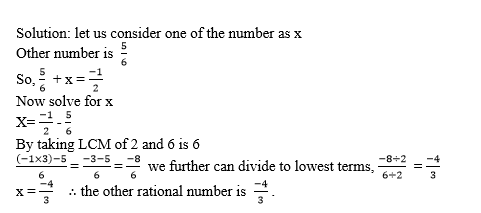Q11. What number should be added to-5/8 so as to get -3/2 ?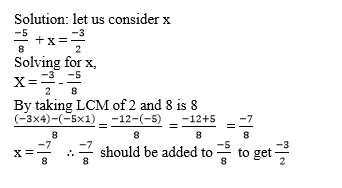Q12. What number should be added to -1 so as to get 5/7 ?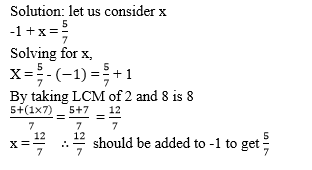Q13. What number should be subtracted from -2/3 to get -1/6 ?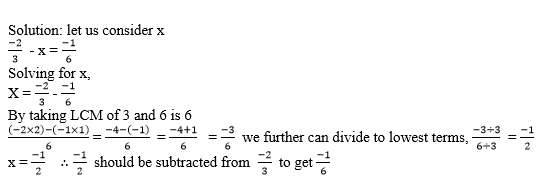Q14.

1. Which rational number is its own additive inverse?
2. Is the difference of two rational numbers a rational number?
3. Is addition commutative on rational numbers?
4. Is addition associative on rational numbers?
5. Is subtraction commutative on rational numbers?
6. Is subtraction associative on rational numbers?
7. What is the negative of a negative rational number?Solutions: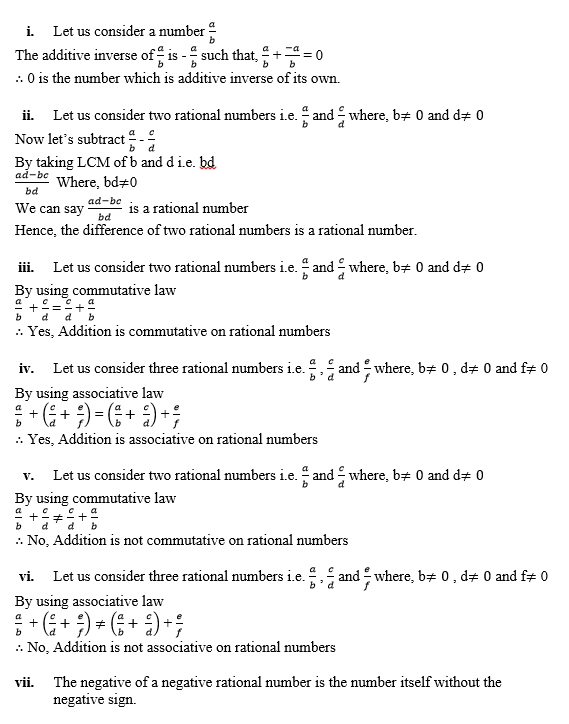### Access other Exercises of RS Aggarwal Solutions for Class 8 Maths Chapter 1- Rational Numbers

Exercise 1A Solutions 10 Questions

Exercise 1B Solutions 3 Questions

Exercise 1D Solutions 9 Questions

Exercise 1E Solutions 12 Questions

Exercise 1F Solutions 8 Questions

Exercise 1G Solutions 20 Questions

Exercise 1H Solutions 23 Questions

## RS Aggarwal Solutions for Class 8 Maths Chapter 1- Rational Numbers Exercise 1C

Exercise 1C of RS Aggarwal Class 8 Chapter 1, Rational Numbers contains the advance concepts related to Rational Numbers. This exercise mainly deals with the Addition and Subtraction of Rational Numbers when their denominators are the same numbers and of unequal numbers. Use of value 0 in the fractions and the Inverse of Rational numbers.

The RS Aggarwal Solutions can help students practice and learn the fundamentals as it provides all the answers to the questions from the RS Aggarwal textbook. By practicing as many times as possible it helps in scoring high marks.